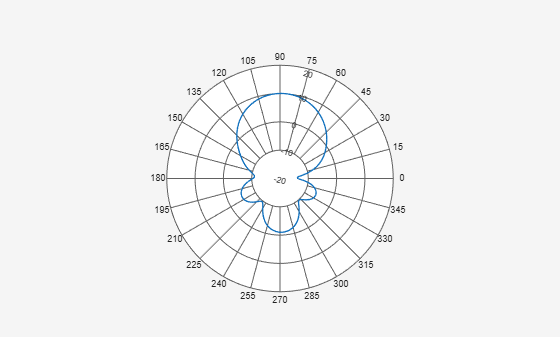# replace

Replace polar plot data with new data

## Syntax

``replace(p,data)``
``replace(p,angle,magnitude)``

## Description

example

````replace(p,data)` removes all data from polar plot, `p` and adds new data based on real amplitude values, `data`. ```
````replace(p,angle,magnitude)` removes all the current data and adds new data sets of angle vectors and corresponding magnitude matrices to the polar plot, `p`.```

## Input Arguments

expand all

Polar plot, specified as a scalar handle.

Antenna or array data, specified as one of the following:

• A real length-M vector, where M contains the magnitude values with angles assumed to be $\frac{\left(0:M-1\right)}{M}×{360}^{\circ }$ degrees.

• A real M-by-N matrix, where M contains the magnitude values and N contains the independent data sets. Each column in the matrix has angles taken from the vector $\frac{\left(0:M-1\right)}{M}×{360}^{\circ }$ degrees. The set of each angle can vary for each column.

• A real N-D array, where N is the number of dimensions. Arrays with dimensions `2` and greater are independent data sets.

• A complex vector or matrix, where `data` contains Cartesian coordinates ((x,y) of each point. x contains the real part of `data` and y contains the imaginary part of `data`.

When data is in a logarithmic form such as dB, magnitude values can be negative. In this case,`polarpattern` plots the lowest magnitude values at the origin of the polar plot and highest magnitude values at the maximum radius.

Set of angles, specified as a vector in degrees.

Set of magnitude values, specified as a vector or a matrix. For a matrix of magnitude values, each column is an independent set of magnitude values and corresponds to the same set of angles.

## Examples

expand all

Create a helix antenna that has a 28 mm radius, a 1.2 mm width, and 4 turns. Calculate the directivity of the antenna at 1.8 GHz.

```hx = helix('Radius',28e-3,'Width',1.2e-3,'Turns',4); H = pattern(hx, 1.8e9,0,0:1:360);```

Plot the polar pattern.

`P = polarpattern(H);`Create a dipole antenna and calculate its directivity at 270 MHz.

```d = dipole; D = pattern(d,270e6,0,0:1:360);```

Replace the existing polar plot of the helix antenna with the directivity of the dipole.

`replace(P,D);`## Version History

Introduced in R2016a